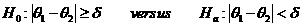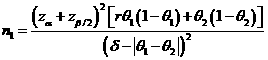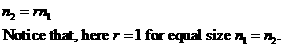# Sample Size Calculator: Two Parallel-Sample Proportions

Hypothesis: One-Sided EquivalenceData Input: (Help) (Example)

 Input Results α β n1 θ1 n2 θ2 N δ r

Note:

 Variables Descriptions α One-sided significance level 1-β Power of the test θ1 Expected success proportions of sample one θ2 Expected success proportions of sample two δ Equivalence limit r Ratio of sample size of group two to group one n1 Sample size of sample one n2 Sample size of sample two N Total Sample size

Help Aids Top

Application: This procedure is used to test the following hypotheses:Procedure:

1. Enter

a)      value of α, the probability of type I error

b)      value of β, the probability of type II error

c)      value of θ1, a true mean response rate of a test drug

d)     value of θ2, the true mean response rates of a control drug

e)      value of δ, the equivalence limit

f)       value of r, allocation ratio, n2/n1.

1. Click the button “Calculate” to obtain result sample size of each group n.

Formula:(*)Notations:

α:               The probability of type I error (significance level) is the probability of rejecting the true null hypothesis.

β:               The probability of type II error (1 – power of the test) is the probability of failing to reject the false null hypothesis.

θ12:         The difference between the true mean response rates of a group1 (i.e., a test drug (θ1) and group2 (i.e., a control (θ2))).

δ:                Clinically meaningful difference. The largest change from the reference value (baseline) that is considered to be trivial.

r:                The allocation ratio, n2/n1. i.e., r=1 for equal allocation.

Example: Suppose it is of interest to establish equivalence of the test drug as compared to the active control agent. Considering of that the true mean cure rates of the treatment agents and the active control are given as θ1=75% and θ2=80%, respectively and the equivalence limit is 20% (i.e., δ=0.2). Then, by (*), the required sample size with equal allocation (r=1) to achieve an 80% power (β=0.2) at α=0.05 can be determined by n1 = n2 = 133

Reference:

1. Casagrande, Pike and Smith (1978), Biometrics 34: 483-486.
2. Chow, Shao and Wang, Sample Size Calculations In Clinical Research, Taylor & Francis, NY. (2003) Pages 88-89.
3. Flesis J.L., Statistical Methods for Rates and Proportions (2nd edition). Wiley: New York, 1981.